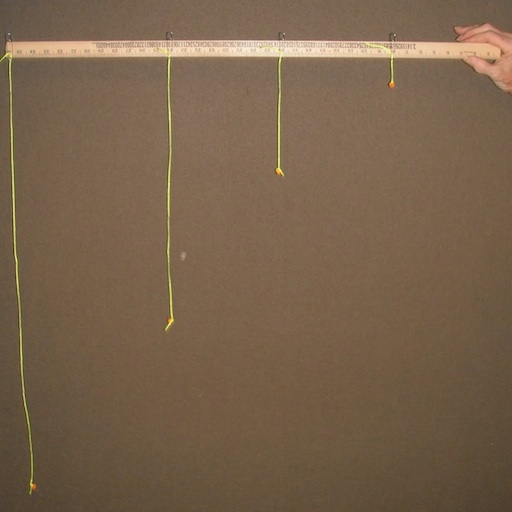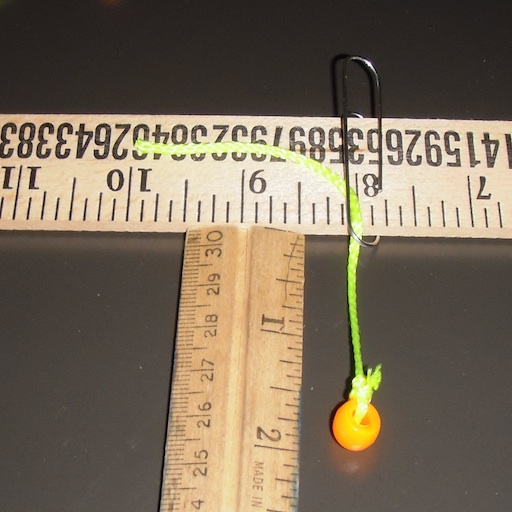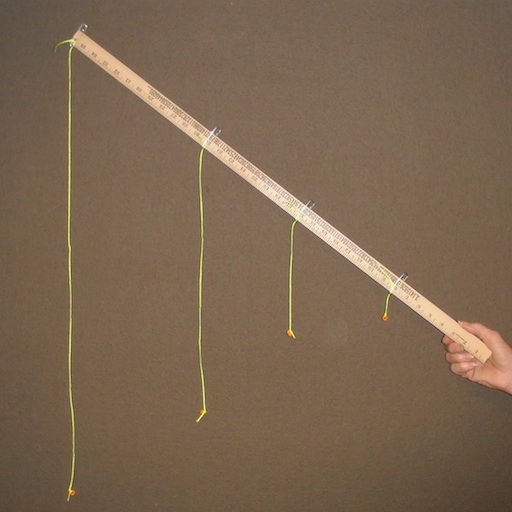String Trajectory

Angling toward understandingIntroduction

You can use beads on pieces of string hanging from a stick to model the trajectory of a particle launched straight and level.

Then tilt the stick at an angle to see the trajectory resulting from launching the particle at an angle.

Material

string, about 2 m colorful is better

A stick, meter stick, yardstick etc. It has to be thin to hold the paperclips.

4 Giant paper clips

A ruler

scissors

To Do and Notice

Mount the paper clips on the stick. put one at 20 cm (8 inches) and others at 40 cm (16 inches) 60 cm (24 inches) and 80 cm (36 inches)

These paper clips are spaced to represent a constant component of velocity along the stick.

The lengths of the strings are in the ratios of 1:4:9:16

Cut the string to lengths of:

5 cm + 10 cm = 15 cm (this length is the fundamental length of 5 cm plus 10 cm to tiee knots at the ends.)

20 cm + 10 cm = 30 cm

45 cm + 10 cm = 55 cm

90 cm + 10 cm = 100 cm

(or 2" + 4" = 6", 12", 22", 36")

Thread the string through the end of the paper clip and pull the loose end behind the paperclip locking it at exactly the right length.What's Going On?

In a trajectory near the surface of the earth follows a parabola.

Constant velocity in the horizontal direction, constant acceleration vertically downward.

Galileo found that if the vertical acceleration produced a motion of 1 unit in 1 time interval, it produced 4 units in 2 time intervals, 9 units in 3 intervals and 16 units in 4 intervals.

We say the distance fallen is proportional to the square of the time.

In metric units where the acceleration of gravity is approximately g = 10 m/s^2 the distance fallen, d, in time, t, is

d = 1/2 g t^2

The great thing about this demo is that it also gives the correct trajectories when the object is launched at an angle upward or downward.Math Root

Galileo knew that if an object fell 1 unit in 1 time interval

it would fall 3 more units in the nest time interval for a sum total of 4 units.

It would fall an additional 5 units in the next time interval for a total of 9 units.

And so on adding sequential odd numbers to produce a series of squares of the integers.

 Scientific Explorations with Paul Doherty © 2013 28 Sep 2013